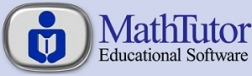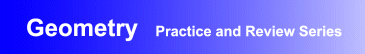Award-Winning

Geometry Interactive Review and Practice Software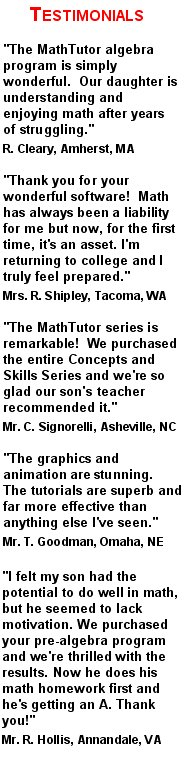DESCRIPTION
GEOMETRY is the perfect

It is

geometry educational software

at its finest.
Parents seeking a homeschool geometry drill and practice instructional software program will find the program to be perfectly suited to their needs.
Educators seeking

curriculum-based geometry practice and review academic instructional software

will find the program to be ideal.
And others who simply want interactive geometry practice and drill computer software designed for self study will find the program to be exactly what they were looking for.
GEOMETRY has been acclaimed for its educational excellence as well as its flexibility. It can be used for self-learning and to supplement classroom instruction in high school and middle school math classes.
Designed by math educators and in accordance with math curriculum standards, the program provides interactive geometry drill and practice exercises suitable for students at all levels of ability.
Examples and geometry problem solving exercises progress gradually from the simpler to the more challenging, allowing students to develop outstanding geometry problem solving skills.
• Award-winning, curriculum-based geometry drill and practice software developed by math educators
• Provides dynamic, interactive geometry problem solving exercises that are ideal for self-study, and as a supplement to classroom instruction
• Demonstrated success in boosting students' math skills and comprehension, and proficiency in problem solving
• Network versions contain student record-keeping, grading, and performance analysis features
AVAILABLE FOR WINDOWS
• Windows XP/Vista
• Windows 7/8
• Windows 10
PRICING Single User Version: \$49.95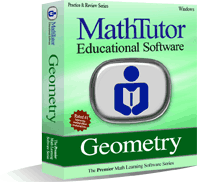TOPICS COVERED
Fundamental Material
• Definitions
• Theorems and Formulas
• Converses
• Constructions
Review of Arithmetic
• Solving an Algebraic Equation
• Fractions: Comparisons
• Fractions: Qualitative
• Arithmetic Comparisons
• Addition and Subtraction of Angles
• Addition and Subtraction of Line Segments
• Angles: Correct Conclusions
• Angles: Incorrect Conclusions
Figures Involving Triangles
• Isosceles Triangles
• Congruent Triangles
• Congruent Angles
• Angle Calculations
• Parallel Lines
• Indirect Proof
• Inequalities in a Triangle
Figures Involving Parallels
• Proportional Lengths
• Angle Comparisons
• Parallelograms
• Coordinates in a Rectangle
• Trapezoids and Right Triangles
• Triangles (connect midpoints); Angles
• Rectangles and Angles
Figures Involving Circles and Polygons
• Proportion: Area, Length, Volume
• Arcs and Angles: Triangle in a Circle
• Arcs and Angles: Circle in a Triangle
• Area Between Two Figures
• Triangles: Midpoints and Areas
• Tangents and Secants
• Historical Summary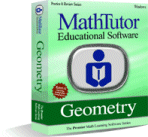Order Now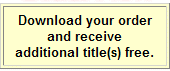TOPICS COVERED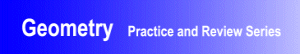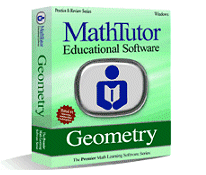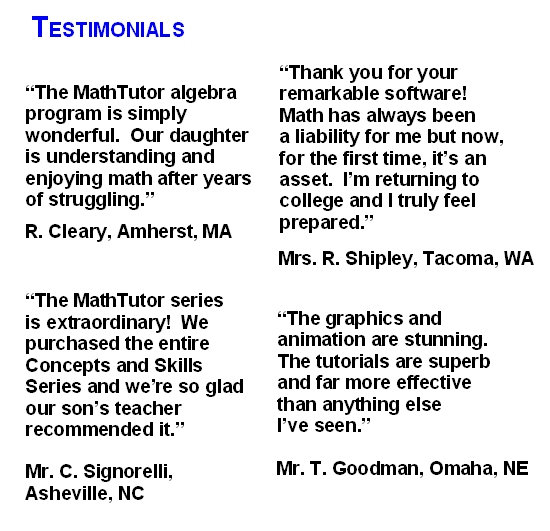TOPICS COVERED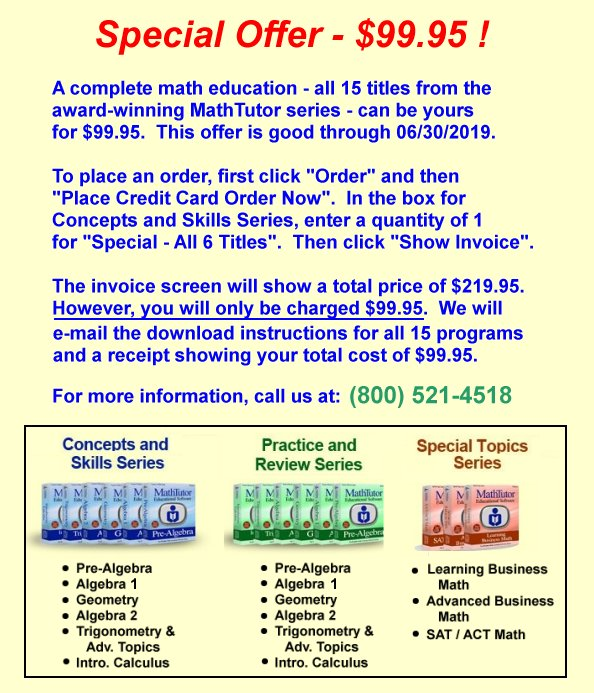×